Open in App
Not now

# Euclid’s Division Algorithm – Real Numbers | Class 10 Maths

• Difficulty Level : Medium
• Last Updated : 07 Nov, 2022

Euclid’s Division Algorithm is a technique to compute the Highest Common Factor (HCF) of two given positive integers. HCF of two positive integers a and b is the largest positive integer d that divides both a and b. To understand Euclid’s Division Algorithm we first need to understand Euclid’s Division Lemma.

### Euclid’s Division Lemma

Euclid’s Division Lemma gives the relation between all the components of Division. Let’s look at the statement of Euclid’s Division Lemma:

For any 2 positive integer a & b there exists a unique integers q & r,

Such that:  a = b x (q + r),

where 0 <= r < b

Let’s understand Euclid’s Division Lemma visually. In the below diagram, the Dividend 27 is a and the Divisor 8 is b.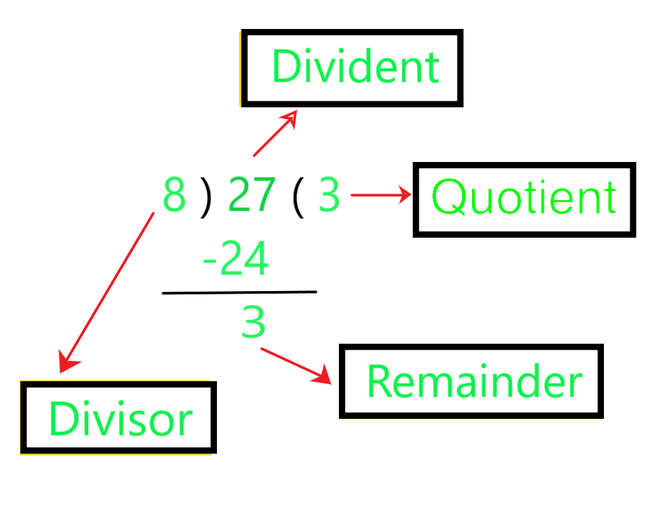The Quotient 3 is q and the Remainder 3 is r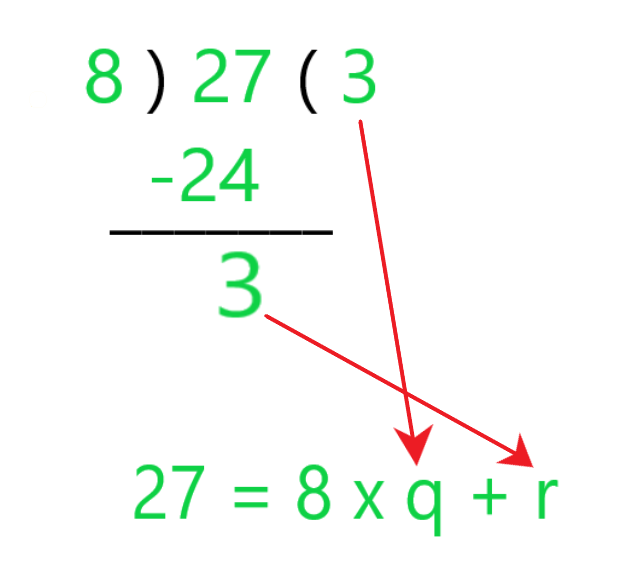As you can see in the below image: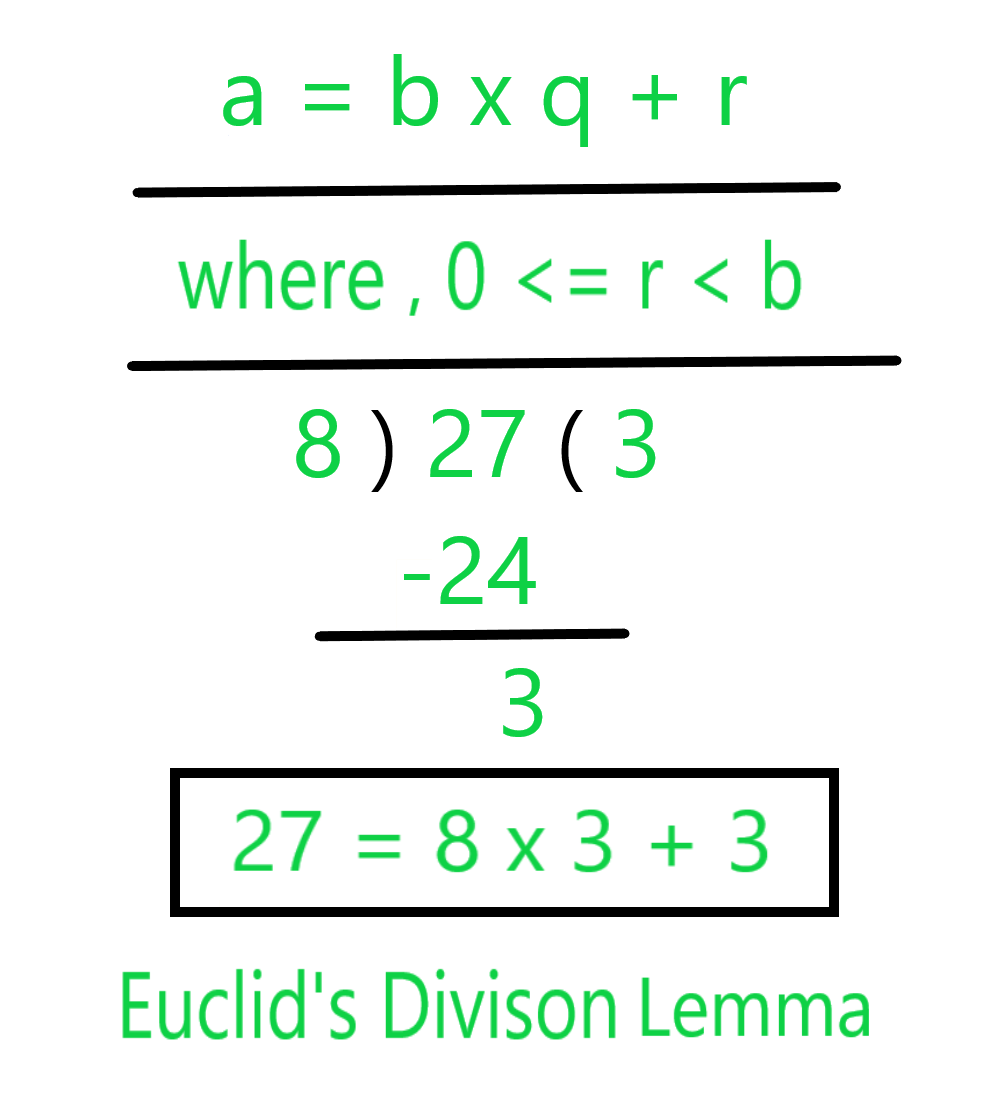Thus, the division lemma: a = b x (q + r) can be written as:

Dividend = (Divisor x Quotient) + Remainder

• Euclid’s Division Lemma has many Applications related to Divisibility of Integers
• It can be used to find the HCF of two numbers.
• HCF  Stands For Highest Common Factor.

### Using Division Lemma to Find the HCF of Two Numbers

The process of finding the HCF of two numbers using EUCLID’S DIVISION LEMMA is called “EUCLID’S DIVISION ALGORITHM”.

Example 1: Let’s find the HCF of 135 and 255?

Solution:

Step 1: Apply the Division Lemma to the 255 and 135, by dividing 255 by 135.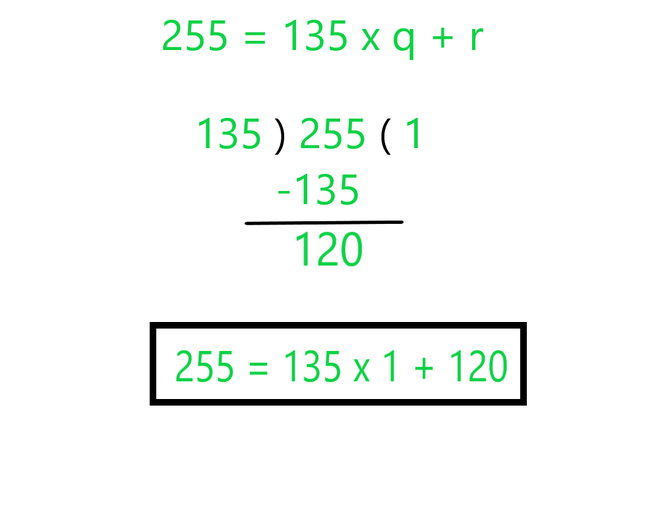Step 2: Now, the Remainder becomes the divisor and the previous divisor becomes the dividend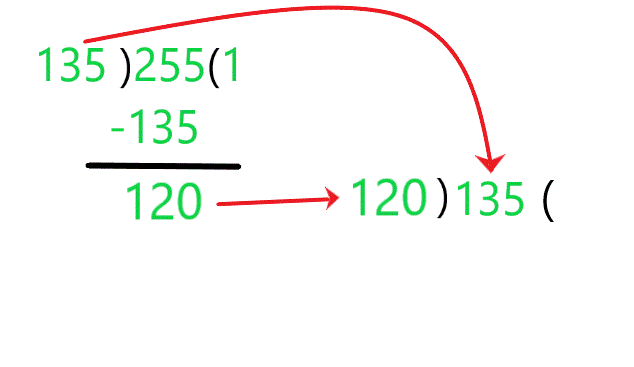Step 3: Again Division Lemma is Applied to this new pair of Dividend and Divisor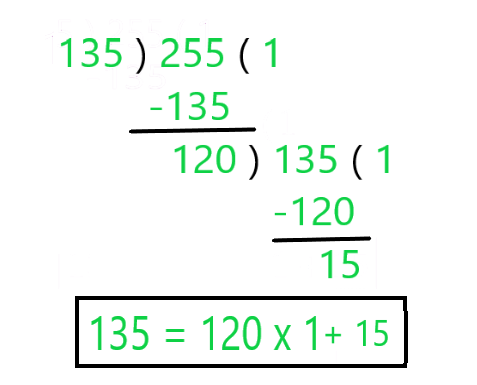Step 4: Now 15 is our new Divisor and 120 is the new Dividend.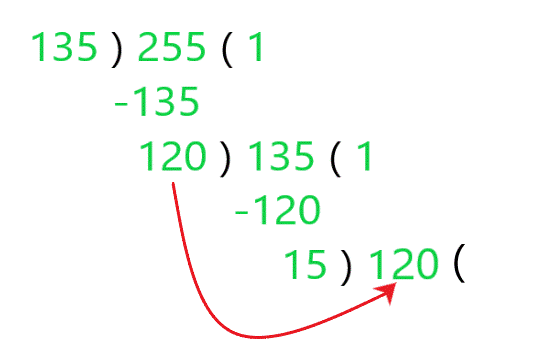The Divisor which makes the Remainder Zero is the HCF of Two Numbers.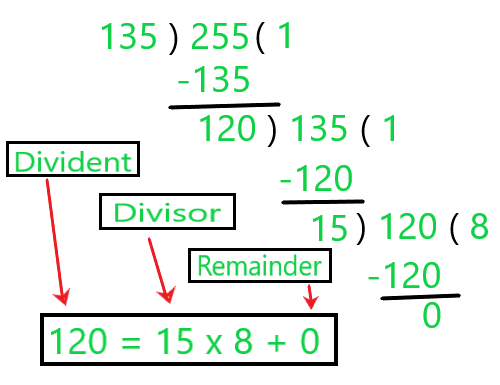So 15 is the HCF of 255 and 135.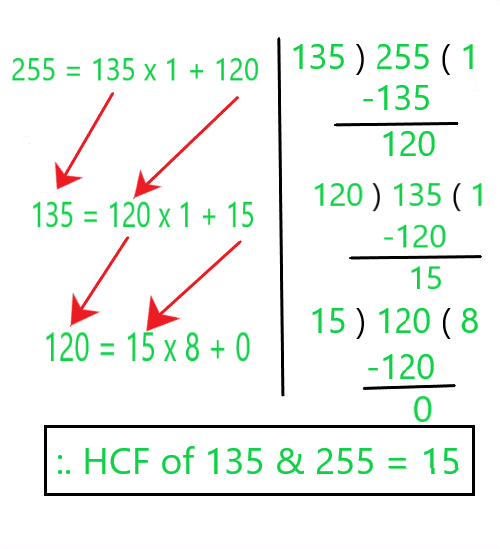Example 2: Use Euclid’s Division Algorithm to Find the HCF of 867 and 255?

Solution:

Step 1:  Since 867 > 255, we apply the Division Lemma to 867 and 255, to get

867  =  255  x  3 + 102

Step 2: Since the Remainder 102 ≠ 0, we apply the division lemma to 867 and  255, to get

255 = 102  x  2  + 51

Step 3: We consider the new divisor 102 and the new Remainder 51 and apply the division lemma to get

102  =  51  x  2 + 0

The remainder has now become zero, so our procedure stops. Since the divisor at this stage is 51, the HCF of 255 and 867  is 51 .

Example 3: Use Euclid’s Division Algorithm to Find the HCF of 4052 and 12576?

Solution:

Step 1: Since 12576 > 4052 , we apply the Division Lemma to 867 and 255, to get

12576  =  4052  x  3 + 420

Step 2: Since the Remainder 420 ≠  0 , we apply the division lemma to 12576 and  4052, to get

4052 = 420  x  9  +  272

Step 3: We consider the new divisor 420 and the new Remainder 272 and apply the division lemma to get

420 =  272  x  1 + 148

We consider the new divisor 272 and the new Remainder 148 and apply the division lemma to get

272  =  148  x  1  + 124

We consider the new divisor 148 and the new Remainder 124 and apply the division lemma to get

148  =  124  x  1  +  24

We consider the new divisor 124 and the new Remainder 24 and apply the division lemma to get

124  =  24  x  5  +  4

We consider the new divisor 24 and the new Remainder 4 and apply the division lemma to get

24 = 4  x  6  +  0

The remainder has now become zero, so our procedure stops . Since the divisor at this stage is 4, the HCF of 4052 and 12576 is 4.

My Personal Notes arrow_drop_up